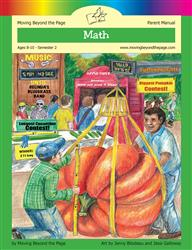# Common Core Alignment

CCSS.Math.Content.4.NF.3.d - Solve word problems involving addition and subtraction of fractions referring to the same whole and having like denominators, e.g., by using visual fraction models and equations to represent the problem.

## 5: MathUnit 5: Fractions
Lesson 13: More Practice and Problem Solving
Lesson 9: Solving Fraction ProblemsUnit 9: Skills Review
Lesson 3: Fractions and Decimals